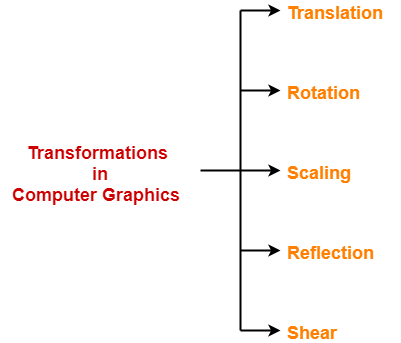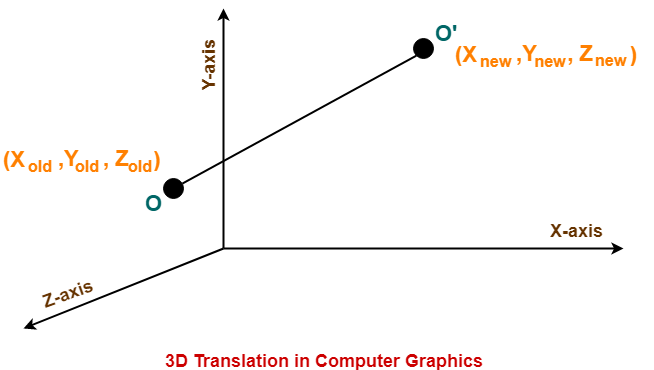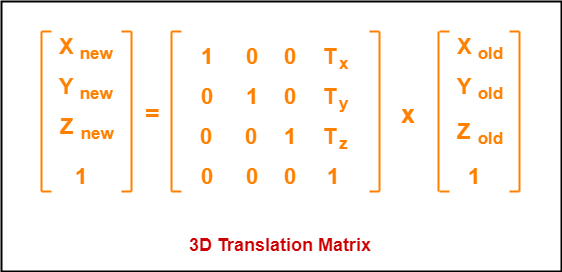## 3D Transformation in Computer Graphics-

 In Computer graphics, Transformation is a process of modifying and re-positioning the existing graphics.

• 3D Transformations take place in a three dimensional plane.
• 3D Transformations are important and a bit more complex than 2D Transformations.
• Transformations are helpful in changing the position, size, orientation, shape etc of the object.

### Transformation Techniques-

In computer graphics, various transformation techniques are-## 3D Translation in Computer Graphics-

 In Computer graphics, 3D Translation is a process of moving an object from one position to another in a three dimensional plane.

Consider a point object O has to be moved from one position to another in a 3D plane.

Let-

• Initial coordinates of the object O = (Xold, Yold, Zold)
• New coordinates of the object O after translation = (Xnew, Ynew, Zold)
• Translation vector or Shift vector = (Tx, Ty, Tz)

Given a Translation vector (Tx, Ty, Tz)-

• Tx defines the distance the Xold coordinate has to be moved.
• Ty defines the distance the Yold coordinate has to be moved.
• Tz defines the distance the Zold coordinate has to be moved.This translation is achieved by adding the translation coordinates to the old coordinates of the object as-

• Xnew = Xold + Tx     (This denotes translation towards X axis)
• Ynew = Yold + Ty     (This denotes translation towards Y axis)
• Znew = Zold + Tz     (This denotes translation towards Z axis)

In Matrix form, the above translation equations may be represented as-Also Read- 2D Translation in Computer Graphics

## Problem-

Given a 3D object with coordinate points A(0, 3, 1), B(3, 3, 2), C(3, 0, 0), D(0, 0, 0). Apply the translation with the distance 1 towards X axis, 1 towards Y axis and 2 towards Z axis and obtain the new coordinates of the object.

## Solution-

Given-

• Old coordinates of the object = A (0, 3, 1), B(3, 3, 2), C(3, 0, 0), D(0, 0, 0)
• Translation vector = (Tx, Ty, Tz) = (1, 1, 2)

### For Coordinates A(0, 3, 1)

Let the new coordinates of A = (Xnew, Ynew, Znew).

Applying the translation equations, we have-

• Xnew = Xold + Tx = 0 + 1 = 1
• Ynew = Yold + Ty = 3 + 1 = 4
• Znew = Zold + Tz = 1 + 2 = 3

Thus, New coordinates of A = (1, 4, 3).

### For Coordinates B(3, 3, 2)

Let the new coordinates of B = (Xnew, Ynew, Znew).

Applying the translation equations, we have-

• Xnew = Xold + Tx = 3 + 1 = 4
• Ynew = Yold + Ty = 3 + 1 = 4
• Znew = Zold + Tz = 2 + 2 = 4

Thus, New coordinates of B = (4, 4, 4).

### For Coordinates C(3, 0, 0)

Let the new coordinates of C = (Xnew, Ynew, Znew).

Applying the translation equations, we have-

• Xnew = Xold + Tx = 3 + 1 = 4
• Ynew = Yold + Ty = 0 + 1 = 1
• Znew = Zold + Tz = 0 + 2 = 2

Thus, New coordinates of C = (4, 1, 2).

### For Coordinates D(0, 0, 0)

Let the new coordinates of D = (Xnew, Ynew, Znew).

Applying the translation equations, we have-

• Xnew = Xold + Tx = 0 + 1 = 1
• Ynew = Yold + Ty = 0 + 1 = 1
• Znew = Zold + Tz = 0 + 2 = 2

Thus, New coordinates of D = (1, 1, 2).

Thus, New coordinates of the object = A (1, 4, 3), B(4, 4, 4), C(4, 1, 2), D(1, 1, 2).

To gain better understanding about 3D Translation in Computer Graphics,

Watch this Video Lecture

Next Article- 3D Rotation in Computer Graphics

Get more notes and other study material of Computer Graphics.

Watch video lectures by visiting our YouTube channel LearnVidFun.